UPSC  >  Test: Alligation & Mixture- 2

# Test: Alligation & Mixture- 2 - UPSC

Test Description

## 10 Questions MCQ Test CSAT Preparation - Test: Alligation & Mixture- 2

Test: Alligation & Mixture- 2 for UPSC 2023 is part of CSAT Preparation preparation. The Test: Alligation & Mixture- 2 questions and answers have been prepared according to the UPSC exam syllabus.The Test: Alligation & Mixture- 2 MCQs are made for UPSC 2023 Exam. Find important definitions, questions, notes, meanings, examples, exercises, MCQs and online tests for Test: Alligation & Mixture- 2 below.
Solutions of Test: Alligation & Mixture- 2 questions in English are available as part of our CSAT Preparation for UPSC & Test: Alligation & Mixture- 2 solutions in Hindi for CSAT Preparation course. Download more important topics, notes, lectures and mock test series for UPSC Exam by signing up for free. Attempt Test: Alligation & Mixture- 2 | 10 questions in 20 minutes | Mock test for UPSC preparation | Free important questions MCQ to study CSAT Preparation for UPSC Exam | Download free PDF with solutions
 1 Crore+ students have signed up on EduRev. Have you?
Test: Alligation & Mixture- 2 - Question 1

### In what ratio must rice at Rs.7.10 be mixed with rice at Rs.9.20 so that the mixture may be worth Rs.8 per Kg?

Test: Alligation & Mixture- 2 - Question 2

### In what ratio must tea at Rs.62 per Kg be mixed with tea at Rs. 72 per Kg so that the mixture must be worth Rs. 64.50 per Kg?

Test: Alligation & Mixture- 2 - Question 3

### A vessel is filled with liquid, 3 parts of which are water and 5 parts of syrup. How much of the mixture must be drawn off and replaced with water so that the mixture may be half water and half syrup?

Detailed Solution for Test: Alligation & Mixture- 2 - Question 3

Let initially vessel have 8 l of liquid and x lof this liquid be replaced with water then quantity of water in new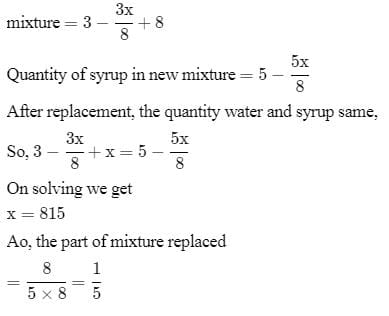Test: Alligation & Mixture- 2 - Question 4

A container contains a mixture of two liquids P and Q in the ratio 7 : 5. When 9 litres of mixture are drawn off and the container is filled with Q, the ratio of P and Q becomes 7 : 9. How many litres of liquid P was contained in the container initially?

Detailed Solution for Test: Alligation & Mixture- 2 - Question 4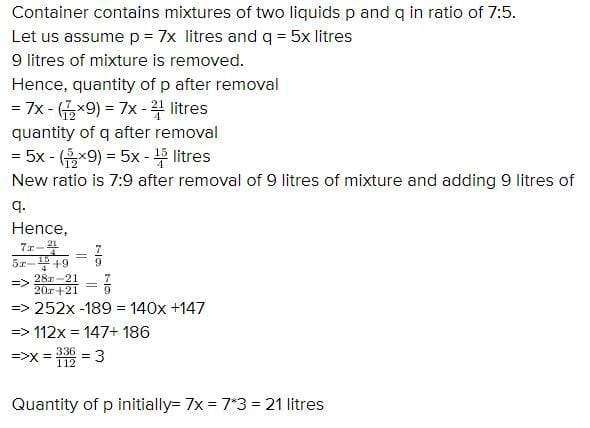Test: Alligation & Mixture- 2 - Question 5

In what ratio must a grocer mix two varieties of pulses costing Rs.15 and Rs. 20 per kg respectively to obtain a mixture worth Rs.16.50 per Kg?

Detailed Solution for Test: Alligation & Mixture- 2 - Question 5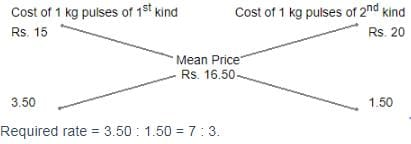Test: Alligation & Mixture- 2 - Question 6

In what ratio must tea worth Rs. 60 per kg be mixed with tea worth Rs. 65 a Kg such that by selling the mixture at Rs. 68.20 a Kg ,there can be a gain 10%?

Detailed Solution for Test: Alligation & Mixture- 2 - Question 6

100/110 × 68.20 = 62
Let the amount of tea worth rs 60 be x and the amount of tea worth rs 65 be y
The total amount is : x + y
60x + 65y = 62(x + y)
60x + 65y = 62x + 62y
65y - 62y = 62x - 60x
3y = 2x
3/2 = x / y
3 : 2

Test: Alligation & Mixture- 2 - Question 7

A merchant has 1000 kg of sugar part of which he sells at 8% profit and the rest at 18% profit. He gains 14% on the whole. The Quantity sold at 18% profit is

Detailed Solution for Test: Alligation & Mixture- 2 - Question 7

Let the sugar sold at 8% gain = x
Therefore, Sugar sold at 18% gain = (1000 - x)
Let CP of sugar = Rs.y per kg
Total CP = Rs.100y
Therefore,
(108/100 * xy) + 118/100(1000-x)y
114/100*1000y
= 108xy + 118000y - 118xy = 114000y
10x = 4000
x = 400
Quantity sold at 18% profit
= 1000 - 400 = 600 kg

Test: Alligation & Mixture- 2 - Question 8

A dishonest milkman sells his milk at cost price but he mixes it with water and thereby gains 25%. What is the percentage of water in the mixture?

Detailed Solution for Test: Alligation & Mixture- 2 - Question 8

Let C.P. of 1 litre milk be Re. 1
Then, S.P. of 1 litre of mixture = Re. 1, Gain = 25%.
C.P. of 1 litre mixture = Re.(100/125 * 1) = 4/5
By the rule of alligation, we have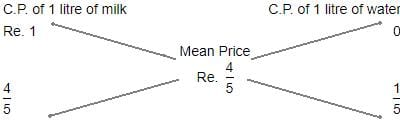Ratio of milk to water = 4/5 : 1/5
=> 4:5
Hence, percentage of water in the mixture = 1/5 x 100%
= 20%

Test: Alligation & Mixture- 2 - Question 9

In what ratio must water be mixed with milk costing Rs.12 per litre in order to get a mixture worth of Rs.8 per litre?

Detailed Solution for Test: Alligation & Mixture- 2 - Question 9

Let's assume cost of water is 0,
And the amount of water in mixture be x litres, and amount of milk in mixture be y litres,
So as per question the mixture would be sold a 8 Rs/litre
=> Cost of water per litre times amount of water in mixture of 1 litre + Cost of milk per litre times amount of milk in mixture of 1 litre must be equal to required cost of 1 litre mixture times 1 litre(total mixture quantity)
Mathematically, 0*x + 12*y = 8*1
=> 12 y = 8
=> y=2/3 litre
Now since total mixture is 1 litre,
So x = 1-y
x = 1-(2/3) = 1/3 litre
So ratio of water to milk in mixture, to get cost of required mixture to 1 litre = x/y = (1/3)/(2/3)
=> Ratio of water to milk in mixture is 1:2

Test: Alligation & Mixture- 2 - Question 10

In what ratio must water be mixed with milk to gain 16 2/3 % on selling the mixture at cost price?

Detailed Solution for Test: Alligation & Mixture- 2 - Question 10

Let C.P. of 1 litre milk be Rs. 1
S.P. of 1 litre of mixture = Rs. 1, Gain =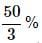∴ C.P. of 1 litre of mixture =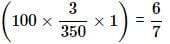BY the rule of alligation, we have: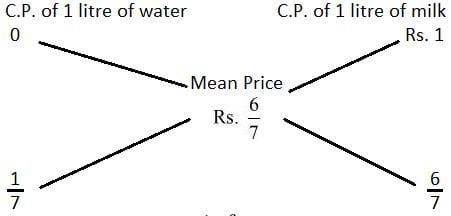∴ Ration of water and milk =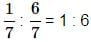## CSAT Preparation

197 videos|151 docs|200 tests
Information about Test: Alligation & Mixture- 2 Page
In this test you can find the Exam questions for Test: Alligation & Mixture- 2 solved & explained in the simplest way possible. Besides giving Questions and answers for Test: Alligation & Mixture- 2, EduRev gives you an ample number of Online tests for practice

## CSAT Preparation

197 videos|151 docs|200 tests

### How to Prepare for UPSC

Read our guide to prepare for UPSC which is created by Toppers & the best Teachers